# Maximize sum of N X N upper left sub-matrix from given 2N X 2N matrix

Given a 2N x 2N matrix of integers. You are allowed to reverse any row or column any number of times and in any order. The task is to calculate the maximum sum of the upper-left N X N submatrix i.e the sum of elements of submatrix from (0, 0) to (N – 1, N – 1).

Examples:

```Input : mat[][] = {
112, 42, 83, 119,
56, 125, 56, 49,
15, 78, 101, 43,
62, 98, 114, 108
}
Output : 414
Given matrix is of size 4 X 4, we need to maximize
the sum of upper left 2 X 2 matrix i.e
the sum of mat + mat + mat + mat.
Following operations maximize the sum:
1. Reverse the column 2,
112, 42, 114, 119,
56, 125, 101, 49,
15, 78, 56, 43,
62, 98, 83, 108

2. Reverse row 0,
119, 114, 42, 112,
56, 125, 101, 49,
15, 78, 56, 43,
62, 98, 83, 108

Sum of upper-left matrix = 119 + 114 + 56 + 125 = 414.
```

## Recommended: Please try your approach on {IDE} first, before moving on to the solution.

To maximize the sum of upper-left submatrix, observe, for each cell of the top-left submatrix, there are four candidates, meaning the corresponding cells in the top-left, top-right, bottom-left, and bottom-right submatrices that it can be swapped with.
Now, observe for each cell, wherever it is, we can swap it with the corresponding candidate value in the top-left submatrix without changing the order of the other cells in the top-left submatrix.The diagram show for an instance where the maximum value of the 4 candidates is in the top-right submatrix. If its is in the bottom-left or bottom-right submatrices, we can first reverse a row or column to put it in the top-right submatrix and then follow the same sequence of operations as shown in diagram.
In this matrix, let say a26 is the maximum of the 4 candidates and a23 must be swapped with a26 without changing the order of the cells in the top-left submatrix.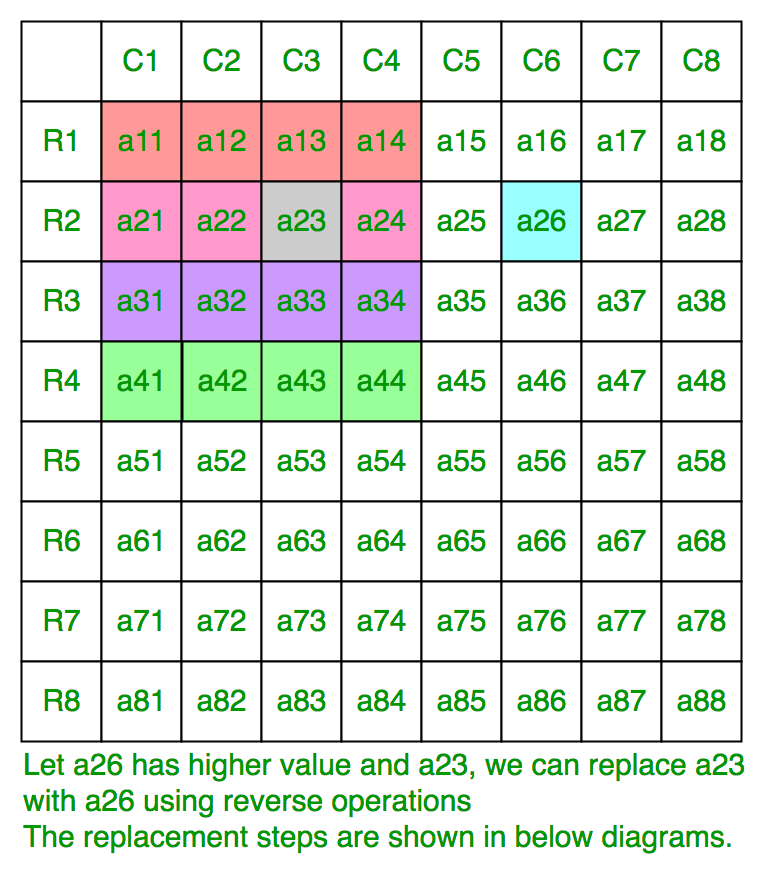Reverse Row 2,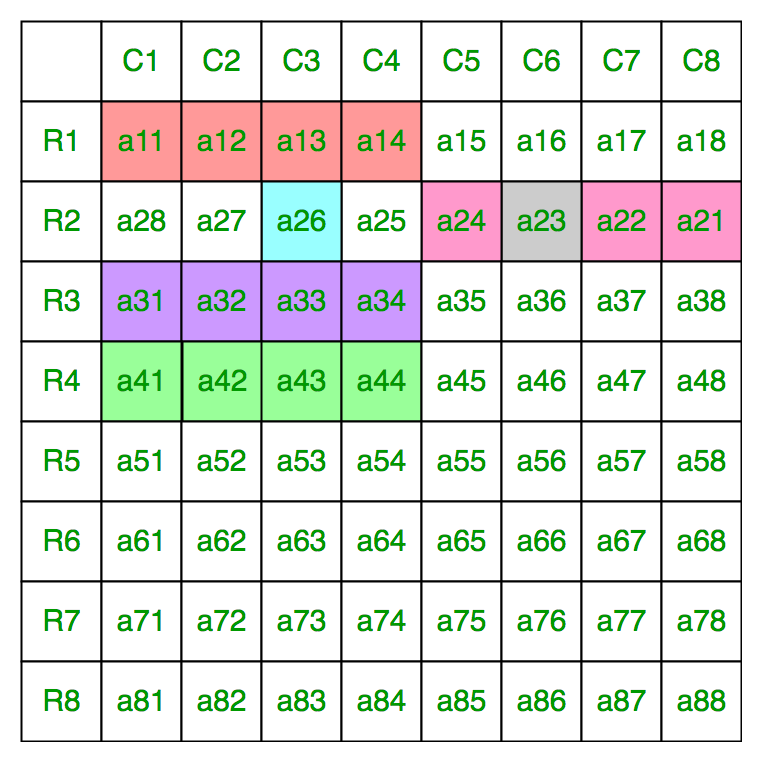Reverse Column 2,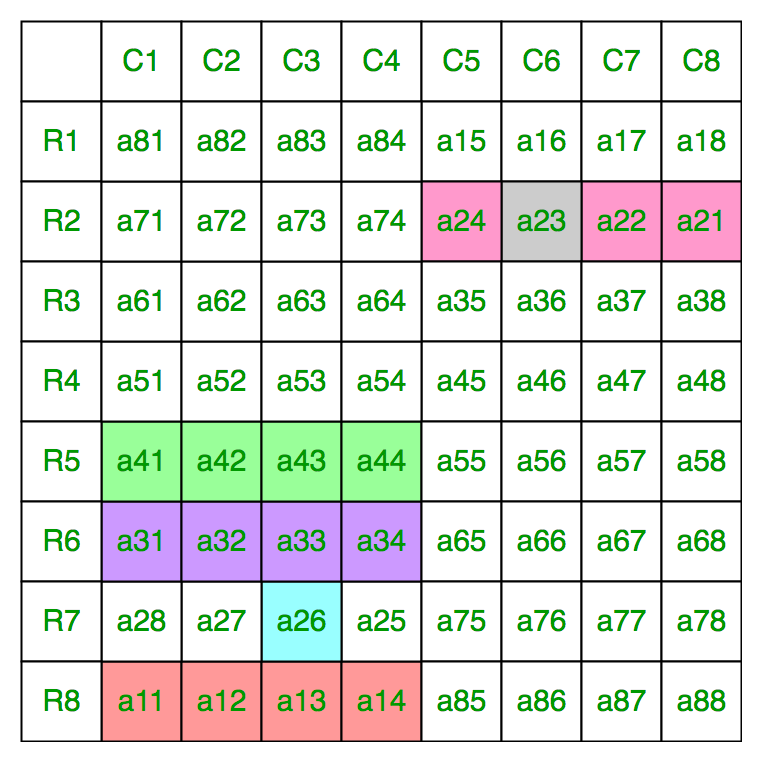Reverse Row 7,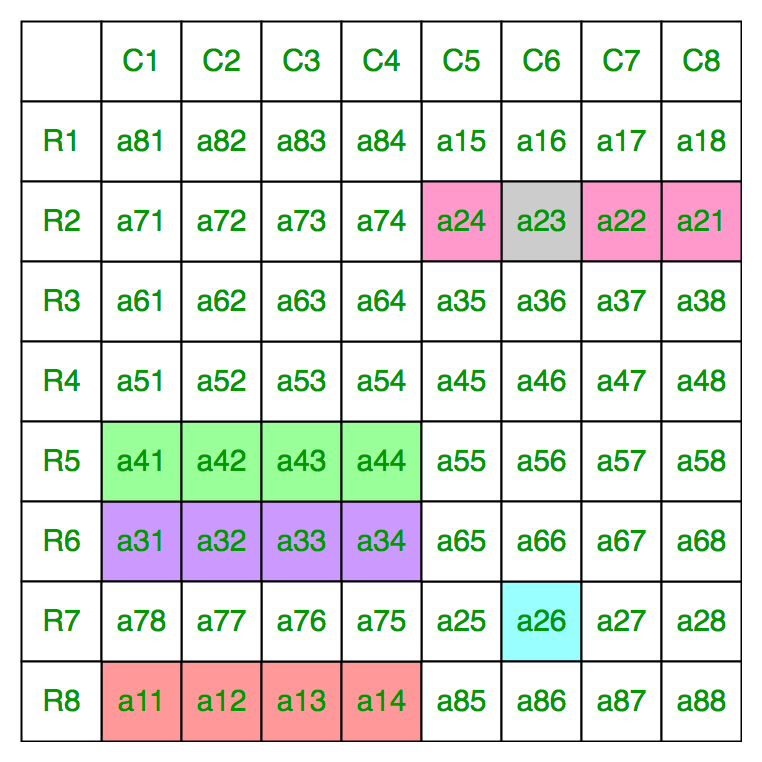Reverse Column 6,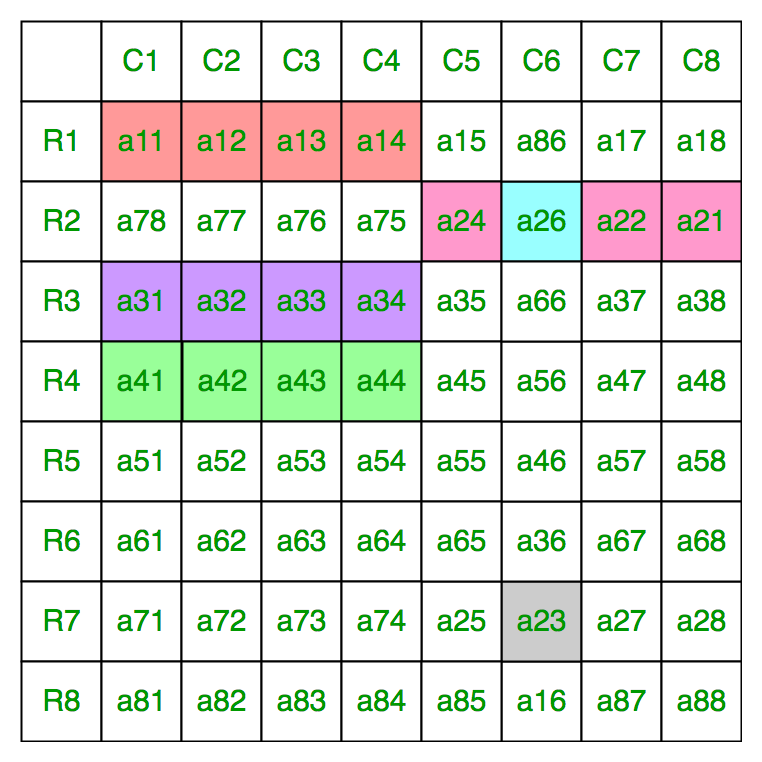Reverse Row 2,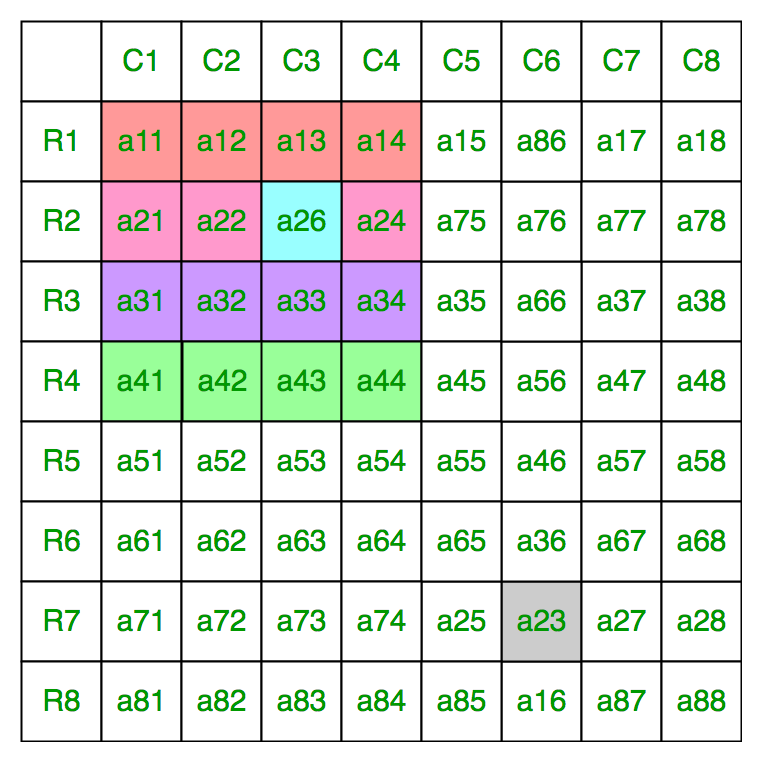Below is the implementation of this approach:

## C++

 `// C++ program to find maximum value of top N/2 x N/2 ` `// matrix using row and column reverse operations ` `#include ` `#define R 4 ` `#define C 4 ` `using` `namespace` `std; ` ` `  `int` `maxSum(``int` `mat[R][C]) ` `{ ` `  ``int` `sum = 0; ` ` `  `  ``for` `(``int` `i = 0; i < R/2; i++) ` `    ``for` `(``int` `j = 0; j < C/2; j++)  ` `    ``{ ` `      ``int` `r1 = i; ` `      ``int` `r2 = R - i - 1; ` `      ``int` `c1 = j; ` `      ``int` `c2 = C - j - 1; ` `       `  `      ``// We can replace current cell [i, j] ` `      ``// with 4 cells without changing affecting ` `      ``// other elements. ` `      ``sum += max(max(mat[r1][c1], mat[r1][c2]),  ` `                 ``max(mat[r2][c1], mat[r2][c2])); ` `    ``} ` `     `  `    ``return` `sum; ` `} ` ` `  `// Driven Program ` `int` `main()  ` `{ ` `  ``int` `mat[R][C] = { ` `                    ``112, 42, 83, 119, ` `                    ``56, 125, 56, 49, ` `                    ``15, 78, 101, 43, ` `                    ``62, 98, 114, 108 ` `                  ``}; ` ` `  `  ``cout << maxSum(mat) << endl; ` ` `  `  ``return` `0; ` `} `

## Java

 `// Java program to find maximum value of top N/2 x N/2 ` `// matrix using row and column reverse operations ` ` `  `class` `GFG { ` ` `  `    ``static` `int` `R = ``4``; ` `    ``static` `int` `C = ``4``; ` ` `  `    ``static` `int` `maxSum(``int` `mat[][]) { ` `        ``int` `sum = ``0``; ` ` `  `        ``for` `(``int` `i = ``0``; i < R / ``2``; i++) { ` `            ``for` `(``int` `j = ``0``; j < C / ``2``; j++) { ` `                ``int` `r1 = i; ` `                ``int` `r2 = R - i - ``1``; ` `                ``int` `c1 = j; ` `                ``int` `c2 = C - j - ``1``; ` ` `  `                ``// We can replace current cell [i, j] ` `                ``// with 4 cells without changing affecting ` `                ``// other elements. ` `                ``sum += Math.max(Math.max(mat[r1][c1], mat[r1][c2]), ` `                        ``Math.max(mat[r2][c1], mat[r2][c2])); ` `            ``} ` `        ``} ` ` `  `        ``return` `sum; ` `    ``} ` ` `  `// Driven Program ` `    ``public` `static` `void` `main(String[] args) { ` `        ``int` `mat[][] = { ` `            ``{``112``, ``42``, ``83``, ``119``}, ` `            ``{``56``, ``125``, ``56``, ``49``}, ` `            ``{``15``, ``78``, ``101``, ``43``}, ` `            ``{``62``, ``98``, ``114``, ``108``} ` `        ``}; ` ` `  `        ``System.out.println(maxSum(mat)); ` ` `  `    ``} ` `} ` `/* This Java code is contributed by Rajput-Ji*/`

## Python3

 `# Python3 program to find the maximum value  ` `# of top N/2 x N/2 matrix using row and  ` `# column reverse operations  ` `def` `maxSum(mat):  ` ` `  `    ``Sum` `=` `0` `    ``for` `i ``in` `range``(``0``, R ``/``/` `2``):  ` `        ``for` `j ``in` `range``(``0``, C ``/``/` `2``):  ` `         `  `            ``r1, r2 ``=` `i, R ``-` `i ``-` `1` `            ``c1, c2 ``=` `j, C ``-` `j ``-` `1` `                 `  `            ``# We can replace current cell [i, j]  ` `            ``# with 4 cells without changing/affecting  ` `            ``# other elements.  ` `            ``Sum` `+``=` `max``(``max``(mat[r1][c1], mat[r1][c2]),  ` `                       ``max``(mat[r2][c1], mat[r2][c2]))  ` `         `  `    ``return` `Sum` ` `  `# Driver Code ` `if` `__name__ ``=``=` `"__main__"``: ` ` `  `    ``R ``=` `C ``=` `4` `    ``mat ``=` `[[``112``, ``42``, ``83``, ``119``],  ` `           ``[``56``, ``125``, ``56``, ``49``],  ` `           ``[``15``, ``78``, ``101``, ``43``],  ` `           ``[``62``, ``98``, ``114``, ``108``]]  ` ` `  `    ``print``(maxSum(mat)) ` ` `  `# This code is contributed  ` `# by Rituraj Jain `

## C#

 `// C# program to find maximum value  ` `// of top N/2 x N/2 matrix using row ` `// and column reverse operations  ` `using` `System; ` ` `  `class` `GFG  ` `{  ` `static` `int` `R = 4;  ` `static` `int` `C = 4;  ` ` `  `static` `int` `maxSum(``int``[,] mat) ` `{  ` `    ``int` `sum = 0;  ` ` `  `    ``for` `(``int` `i = 0; i < R / 2; i++)  ` `    ``{  ` `        ``for` `(``int` `j = 0; j < C / 2; j++) ` `        ``{  ` `            ``int` `r1 = i;  ` `            ``int` `r2 = R - i - 1;  ` `            ``int` `c1 = j;  ` `            ``int` `c2 = C - j - 1;  ` ` `  `            ``// We can replace current cell [i, j]  ` `            ``// with 4 cells without changing affecting  ` `            ``// other elements.  ` `            ``sum += Math.Max(Math.Max(mat[r1,c1], mat[r1,c2]),  ` `                            ``Math.Max(mat[r2,c1], mat[r2,c2]));  ` `        ``}  ` `    ``}  ` `    ``return` `sum;  ` `}  ` ` `  `// Driven Code ` `public` `static` `void` `Main() ` `{  ` `    ``int``[,] mat = ` `    ``{  ` `        ``{112, 42, 83, 119},  ` `        ``{56, 125, 56, 49},  ` `        ``{15, 78, 101, 43},  ` `        ``{62, 98, 114, 108}  ` `    ``};  ` ` `  `    ``Console.Write(maxSum(mat));  ` `}  ` `}  ` ` `  `// This code is contributed ` `// by ChitraNayal `

## PHP

 ` `

Output:

```414
```

Time Complexity: O(N2).

This article is contributed by Anuj Chauhan. If you like GeeksforGeeks and would like to contribute, you can also write an article using contribute.geeksforgeeks.org or mail your article to contribute@geeksforgeeks.org. See your article appearing on the GeeksforGeeks main page and help other Geeks.

My Personal Notes arrow_drop_up

Article Tags :
Practice Tags :

Be the First to upvote.

Please write to us at contribute@geeksforgeeks.org to report any issue with the above content.